×#### Thank you for registering.

One of our academic counsellors will contact you within 1 working day.

Click to Chat

1800-1023-196

+91-120-4616500

CART 0

• 0

MY CART (5)

Use Coupon: CART20 and get 20% off on all online Study Material

ITEM
DETAILS
MRP
DISCOUNT
FINAL PRICE
Total Price: Rs.

There are no items in this cart.
Continue Shopping• Complete JEE Main/Advanced Course and Test Series
• OFFERED PRICE: Rs. 15,900
• View Details

```Mensuration II (Volumes and Surface Areas of a Cuboid and a Cube) Exercise 21.4

Question: 1

Find the length of the longest rod that can be placed in a room 12 m long, 9 m broad and 8 m high.

Solution:Question: 2

If V is the volume of a cuboid of dimension a, b , c and S is its surface area, then prove that:Solution: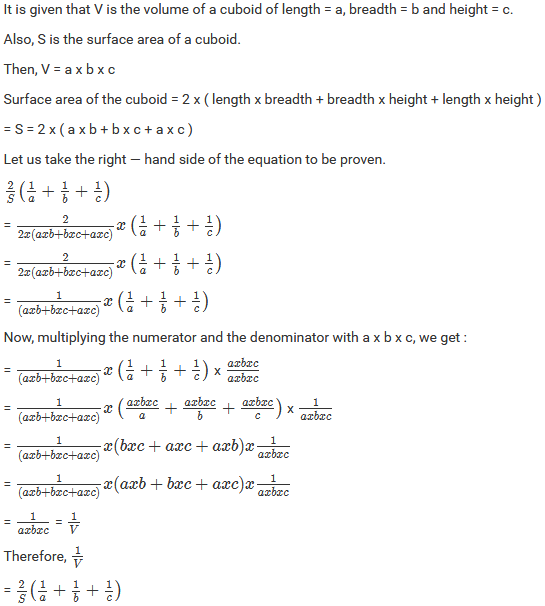Question: 3

The area of three adjacent faces of a cuboid are x, y and z. If the volume is V, Prove that V 2 = x y z.

Solution: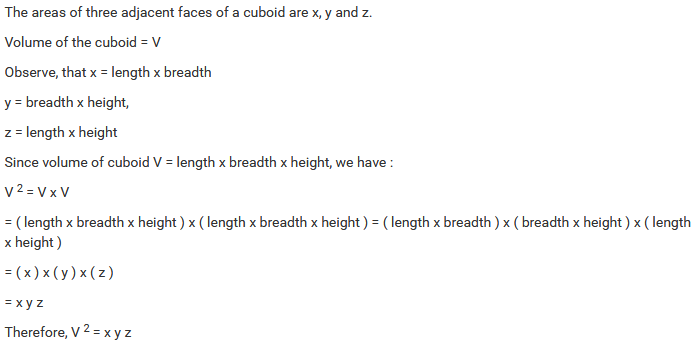Question: 4

A rectangular water reservoir contains 105 m 3 of water. Find the depth of the water in the reservoir if its base measures 12 m by 3.5 m.

Solution:Question: 5

Cubes A, B, C, having edges 18 cm, 24 cm, and 30 cm respectively are melted and molded into a new cube D. Find the edge and bigger cube D.

Solution: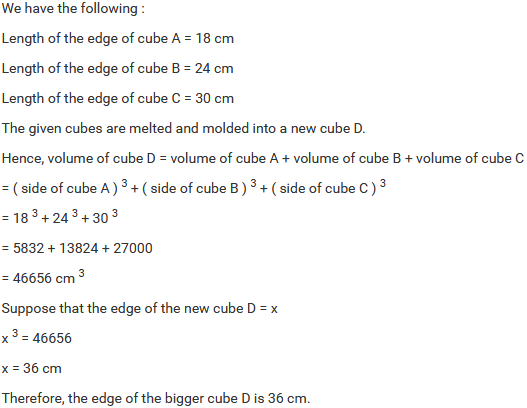Question: 6

The breadth of a room is twice its height, one half of its length and the volume of the room is 512 cu Dm. Find its dimension.

Solution: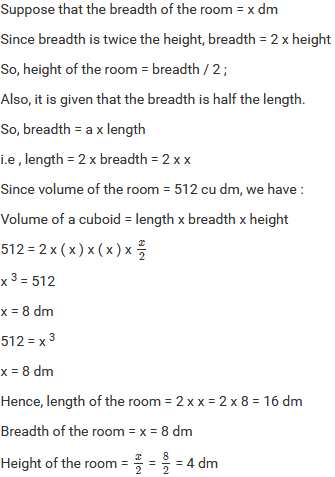Question: 7

A closed iron tank 12 m long, 9m wide and 4 m deep is to be made. Determine the cost of an iron sheet used at the rate of Rs. 5 per meter sheet, the sheet being 2 m wide.

Solution:Question: 8

A tank open at the top is made of an iron sheet 4 m wide. If the dimensions of the tank are 12 m x 8 m x 6 m, find the cost of an iron sheet at Rs. 17.50 per meter.

Solution:Question: 9

Three equal cubes are placed adjacently in a row. Find the ratio of the total surface area of the new cuboid to that of the sum of the surface areas of the three cubes.

Solution:Question: 10

The dimensions of a room are 12.5 m by 9 m by 7 m. there are 2 doors and 4 windows in the room; each door measures 2.5 m by 1.2 m and each window 1.5 m by 1 m. Find the cost of painting the walls at Rs. 3.50 per square meter.

Solution:Question: 11

A field is 150 m long and 100 m wide. A plot ( outside the field ) 50 m long and 30 m wide is dug to a depth of 8 m and the earth taken out from the plot is spread evenly in the field. By how much is the level of field raised?

Solution:Question: 12

Two cubes, each of volume 512 cm 3 are joined end to end. Find the surface area of the resulting cuboid.

Solution: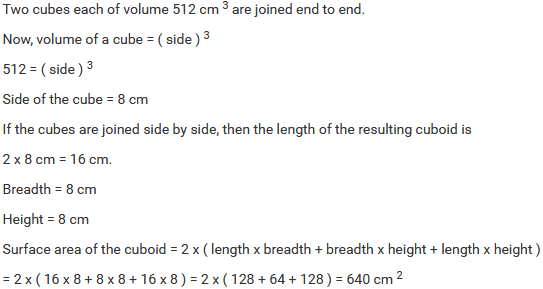Question: 13

Three cubes, each whose edges measure 3 cm, 4 cm and 5 cm respectively are melted to form a new cube. Find the surface area of the new cube formed.

Solution:Question: 14

The cost of preparing the walls of a room 12 m long at the rate of Rs. 1.35 per square meter is Rs. 304.20 and the cost of matting the floor at 85 paise per square meter is Rs. 91.80. Find the height of the room.

Solution:Question: 15

The length of a hall is 18 m and the width 12 m. the sum of the area of the floor and the flat roof is equal to the sum of the area of the four walls. Find the height of the wall.

Solution: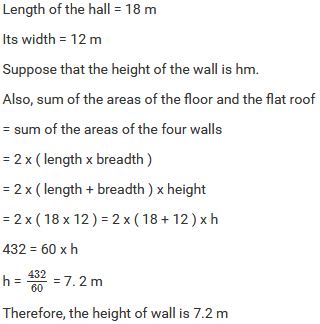Question: 16

A metal cube edge 12 cm is melted and formed into three smaller cubes. If the edges of the two smaller cubes are 6 cm and 8 cm, find the edge of the third smaller cube.

Solution:Question: 17

The dimensions of a cinema hall are 100 m, 50 m and 18 m. How many people can sit in the hall, if each person requires 150 m3 of air?

Solution:Question: 18

The external dimensions of a closed wooden box are 48 cm, 36 cm, 30 cm. the box is made of 1.5 cm thick wood. How many bricks of size 6 cm x 3 cm x 0.75 cm can be put in this box?

Solution:Question: 19

The dimensions of a rectangular box are in the ratio of 2 : 3 : 4 and the difference between the cost of covering it with the sheet of paper at the rates of Rs. 8 and Rs . 9.50 per m 2 is Rs. 1248. Find the dimensions of the box.

Solution:```### Course Features

• 728 Video Lectures
• Revision Notes
• Previous Year Papers
• Mind Map
• Study Planner
• NCERT Solutions
• Discussion Forum
• Test paper with Video Solution